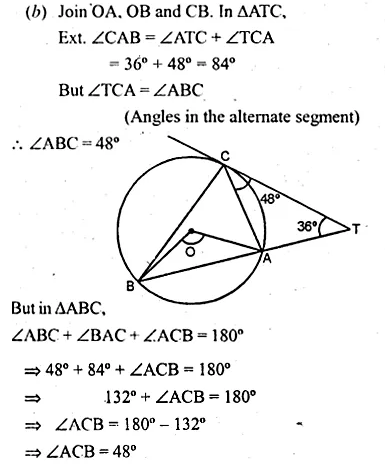Guru

# Ques 35(b) In the figure (ii) given below, A, B and C are three points on a circle. The tangent at C meets BA produced at T. Given that ∠ATC = 36° and ∠ACT = 48°, calculate the angle subtended by AB at the centre of the circle.

• 0

One of the most important and exam oriented question from Chapter name- circles
Topic – Angle properties of circles
Chapter number- 15

This ques has been asked im 2001 exam.

In this question we have a figure in which ot it given that A, B and C are three points on a circle.

The tangent at C meets BA produced at T.

Also we have  that ∠ATC = 36° and ∠ACT = 48°,

Now we have to calculate the angle subtended by AB at the centre of the circle.

ICSE Avichal publication
Understanding ICSE Mathematics
Question 35(b)

Share

1.